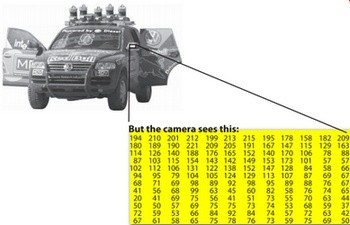### 字节与字节数组

0x07 中介绍了 Python 中的字符串类型，字符串类型是对人类友好的符号，但计算机只认识一种符号，那就是二进制（binary）数，或者说是数字：The core built-in types for manipulating binary data are `bytes` and `bytearray`.

### 1Byte of ASCII``````print(b"Python")
python = (b'P' b'y' b"t" b'o' b'n')
print(python)
``````
``````b'Python'
b'Pyton'
``````

Bytes 代表的是（二进制）数字的序列，只不过在是通过 `ASCII` 编码之后才是我们看到的字符形式，如果我们单独取出一个字节，它仍然是一个数字：

``````print(b"Python")
``````
``````80
``````

``````print(b"雨")
``````
``````  File "<ipython-input-3-1f95d71c6553>", line 1
print(b"雨")
^
SyntaxError: bytes can only contain ASCII literal characters.
``````

0~127~255

``````print(b'\xff')
print(b'\x24')
``````
``````255
b'\$'
``````

``````print(bytes())
print(bytes([36,36,36])) # 记住字节类型是一个序列
``````
``````b'\x18'
b'\$\$\$'
``````

``````print(bytes.fromhex("7b 7d"))

# 逆运算
print(b'{ }'.hex())

int(b' '.hex(), base=16)
``````
``````b'{}'
7b207d

32
``````

### `encode`

``````print("\$".encode('ascii'))
print("\$".encode('ascii'))
``````
``````b'\$'
36
``````

``````snake = '🐍'
try:
snake.encode('ascii')
except UnicodeEncodeError as err:
print(err)

# 正确的做法应该是用 UTF-8 进行编码，因为字符串都是 UTF-8 的
print(snake.encode()) # utf-8 by default
``````
``````'ascii' codec can't encode character '\U0001f40d' in position 0: ordinal not in range(128)
b'\xf0\x9f\x90\x8d'
``````

### 字节数组

``````ba = bytearray(b'hello')
ba[0:1] = b'w'
print(ba)
``````
``````bytearray(b'wello')
``````

### 总结

1. 字节（字节数组）是二进制数据组成的序列，其中每个元素由8bit二进制即1byte亦即2位十六进制数亦亦即0~255组成；
2. 字节是计算机的语言，字符串是人类语言，它们之间通过编码表形成一一对应的关系；
3. 最小的 `ASCII` 编码表只需要一位字节，且只占用了其中 `[31,127]` 的码位；